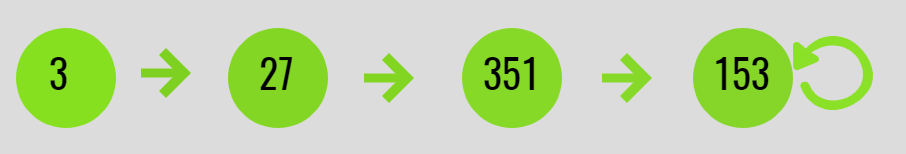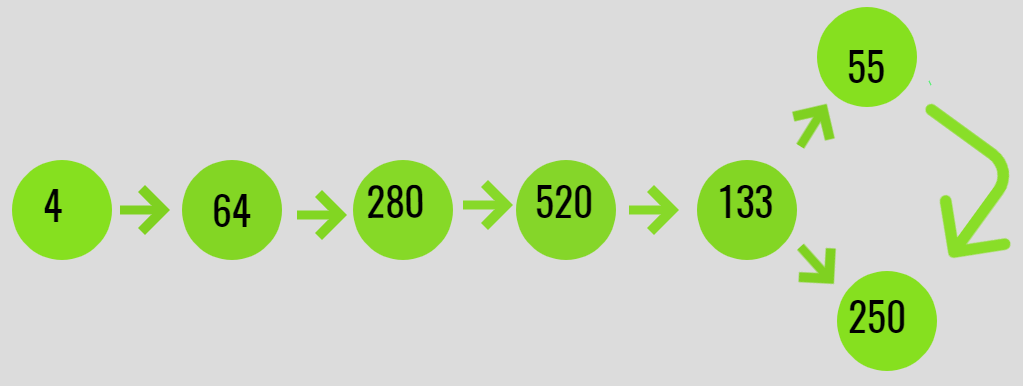# Check if digit cube limit of an integer arrives at fixed point or a limit cycle

Given an integer N, the task is to check if the Digit Cube limit of an integer arrives at a fixed point or in a limit cycle.

A Digit Cube Limit is a number which repeatedly arrives at a point if its value is computed as the sum of cubes of its digits, i.e. they have the following properties:

• Arrive at a fixed point if it is an Armstrong number.
• Arrive at a limit cycle if it is repeating in a cycle.

Examples:

Input: N = 3
Output: Reached to fixed point 153
Explanation:
F(3) = 3 * 3 * 3 = 27
F(27) = 2*2*2 + 7*7*7 = 351
F(351) = 3*3*3 + 5*5*5 + 1*1*1 = 153
F(153) = 1*1*1 + 5*5*5 + 3*3*3 = 153
Since a fixed point(= 153) was obtained, which is an Armstrong number of order 3. Below is the illustration:Input: N = 4
Output: Arrived at cycle
Explanation:
F(4) = 4 * 4 * 4 = 64
F(64) = 6 * 6 * 6 + 4 * 4 * 4 = 280
F(280) = 2 * 2 * 2 + 8 * 8 * 8 + 0 * 0 * 0 = 520
F(520) = 5 * 5 * 5 + 2 * 2 * 2 + 0*0*0 = 133
F(133) = 1*1*1 + 3*3*3 + 3*3*3 = 55
F(55) = 5*5*5 + 5*5*5 = 250
F(250) = 5*5*5 + 2*2*2 + 0*0*0 = 133
A cycle between
133 -> 55 -> 250 -> 133 is obtained.
Below is the illustration of the same:Approach: Follow the steps below to solve the problem:

• Create a hashMap to store the sum of the cube of digits of the number while iteration.
• Iterate for the next values for the sum of cube of digits of a number and Check if the next sum of the cube of digits is already present in the hashMap or not.
• If the number is already in the hash-map then, check if the number is an Armstrong number or not. If found to be true, then the number reaches a fixed point.
• Otherwise, If the number is not Armstrong number then continue.

Below is the implementation of the above approach:

## C++

 `// C++ program for the above approach`   `#include ` `#include `   `// Define the limit` `#define limit 1000000000`   `using` `namespace` `std;`   `// Function to get the sum of cube` `// of digits of a number` `long` `long` `F(``long` `long` `N)` `{` `    ``// Convert to string to get sum` `    ``// of the cubes of its digits` `    ``string str = to_string(N);` `    ``long` `long` `sum = 0;`   `    ``for` `(``long` `long` `i = 0;` `         ``i < str.size(); i++) {` `        ``long` `long` `val` `            ``= ``int``(str[i] - ``'0'``);` `        ``sum += val * val * val;` `    ``}`   `    ``// return sum` `    ``return` `sum;` `}`   `// Function to check if the number` `// arrives at a fixed point or a cycle` `long` `long` `findDestination(``long` `long` `N)` `{` `    ``// Stores the values obtained` `    ``set<``long` `long``> s;`   `    ``long` `long` `prev = N, next;`   `    ``// Insert N to set s` `    ``s.insert(N);`   `    ``while` `(N <= limit) {`   `        ``// Get the next number using F(N)` `        ``next = F(N);`   `        ``// Check if the next number is` `        ``// repeated or not` `        ``auto` `it = s.find(next);` `        ``if` `(it != s.end()) {` `            ``return` `next;` `        ``}`   `        ``prev = next;` `        ``s.insert(prev);` `        ``N = next;` `    ``}` `    ``return` `next;` `}`   `// Function to check if digit cube` `// limit of an integer arrives at` `// fixed point or in a limit cycle` `void` `digitCubeLimit(``long` `long` `N)` `{`   `    ``// N is a non negative integer` `    ``if` `(N < 0)` `        ``cout << ``"N cannot be negative\n"``;`   `    ``else` `{`   `        ``// Function Call` `        ``long` `long` `ans` `            ``= findDestination(N);`   `        ``// If the value received is` `        ``// greater than limit` `        ``if` `(ans > limit)` `            ``cout << ``"Limit exceeded\n"``;`   `        ``// If the value received is` `        ``// an Armstrong number` `        ``else` `if` `(ans == F(ans)) {` `            ``cout << N;` `            ``cout << ``" reaches to a"` `                 ``<< ``" fixed point: "``;` `            ``cout << ans;` `        ``}`   `        ``else` `{` `            ``cout << N;` `            ``cout << ``" reaches to a"` `                 ``<< ``" limit cycle: "``;` `            ``cout << ans;` `        ``}` `    ``}` `}`   `// Driver Code` `int` `main()` `{` `    ``long` `long` `N = 3;`   `    ``// Function Call` `    ``digitCubeLimit(N);`   `    ``return` `0;` `}`

## Java

 `// Java program for the` `// above approach` `import` `java.util.*;` `class` `GFG{` `    `  `// Define the limit` `static` `final` `int` `limit = ``1000000000``;` `  `  `// Function to get the sum of cube` `// of digits of a number` `static` `int` `F(``int` `N)` `{` `  ``// Convert to String to get sum` `  ``// of the cubes of its digits` `  ``String str = String.valueOf(N);` `  ``int` `sum = ``0``;`   `  ``for` `(``int` `i = ``0``;` `           ``i < str.length(); i++) ` `  ``{` `    ``int` `val = (``int``)(str.charAt(i) - ``'0'``);` `    ``sum += val * val * val;` `  ``}`   `  ``// return sum` `  ``return` `sum;` `}`   `// Function to check if the number` `// arrives at a fixed point or a cycle` `static` `int` `findDestination(``int` `N)` `{` `  ``// Stores the values obtained` `  ``HashSet s = ``new` `HashSet<>();`   `  ``int` `prev = N, next =``0``;`   `  ``// Insert N to set s` `  ``s.add(N);`   `  ``while` `(N <= limit) ` `  ``{` `    ``// Get the next number ` `    ``// using F(N)` `    ``next = F(N);`   `    ``// Check if the next number is` `    ``// repeated or not` `    ``if` `(s.contains(next)) ` `    ``{` `      ``return` `next;` `    ``}`   `    ``prev = next;` `    ``s.add(prev);` `    ``N = next;` `  ``}` `  ``return` `next;` `}`   `// Function to check if digit cube` `// limit of an integer arrives at` `// fixed point or in a limit cycle` `static` `void` `digitCubeLimit(``int` `N)` `{` `  ``// N is a non negative integer` `  ``if` `(N < ``0``)` `    ``System.out.print(``"N cannot be negative\n"``);` `  ``else` `  ``{` `    ``// Function Call` `    ``int` `ans = findDestination(N);`   `    ``// If the value received is` `    ``// greater than limit` `    ``if` `(ans > limit)` `      ``System.out.print(``"Limit exceeded\n"``);`   `    ``// If the value received is` `    ``// an Armstrong number` `    ``else` `if` `(ans == F(ans)) ` `    ``{` `      ``System.out.print(N);` `      ``System.out.print(``" reaches to a"` `+ ` `                       ``" fixed point: "``);` `      ``System.out.print(ans);` `    ``}`   `    ``else` `    ``{` `      ``System.out.print(N);` `      ``System.out.print(``" reaches to a"` `+ ` `                       ``" limit cycle: "``);` `      ``System.out.print(ans);` `    ``}` `  ``}` `}`   `// Driver Code` `public` `static` `void` `main(String[] args)` `{` `  ``int` `N = ``3``;`   `  ``// Function Call` `  ``digitCubeLimit(N);` `}` `}`   `// This code is contributed by Rajput-Ji`

## Python3

 `# Python3 program for the above approach`   `# Define the limit` `LIMIT ``=` `1000000000`   `# Function to get the sum of cube` `# of digits of a number` `def` `F(N: ``int``) ``-``> ``int``:` `    `  `    ``# Convert to string to get sum` `    ``# of the cubes of its digits` `    ``string ``=` `str``(N)` `    ``sum` `=` `0` `    `  `    ``for` `i ``in` `range``(``len``(string)):` `        ``val ``=` `int``(``ord``(string[i]) ``-` `ord``(``'0'``))` `        ``sum` `+``=` `val ``*` `val ``*` `val`   `    ``# Return sum` `    ``return` `sum`   `# Function to check if the number` `# arrives at a fixed point or a cycle` `def` `findDestination(N: ``int``) ``-``> ``int``:` `    `  `    ``# Stores the values obtained` `    ``s ``=` `set``()`   `    ``prev ``=` `N` `    ``next` `=` `0`   `    ``# Insert N to set s` `    ``s.add(N)`   `    ``while` `(N <``=` `LIMIT):`   `        ``# Get the next number using F(N)` `        ``next` `=` `F(N)`   `        ``# Check if the next number is` `        ``# repeated or not` `        ``if` `next` `in` `s:` `            ``return` `next`   `        ``prev ``=` `next` `        ``s.add(prev)` `        ``N ``=` `next`   `    ``return` `next`   `# Function to check if digit cube` `# limit of an integer arrives at` `# fixed point or in a limit cycle` `def` `digitCubeLimit(N: ``int``) ``-``> ``int``:` `    `  `    ``# N is a non negative integer` `    ``if` `(N < ``0``):` `        ``print``(``"N cannot be negative"``)`   `    ``else``:`   `        ``# Function Call` `        ``ans ``=` `findDestination(N)`   `        ``# If the value received is` `        ``# greater than limit` `        ``if` `(ans > LIMIT):` `            ``print``(``"Limit exceeded"``)`   `        ``# If the value received is` `        ``# an Armstrong number` `        ``elif` `(ans ``=``=` `F(ans)):` `            ``print``(``"{} reaches to a fixed point: {}"``.``format``(` `                ``N, ans))` `        ``else``:` `            ``print``(``"{} reaches to a limit cycle: {}"``.``format``(` `                ``N, ans))`   `# Driver Code` `if` `__name__ ``=``=` `"__main__"``:` `    `  `    ``N ``=` `3`   `    ``# Function Call` `    ``digitCubeLimit(N)`   `# This code is contributed by sanjeev2552`

## C#

 `// C# program for the` `// above approach` `using` `System;` `using` `System.Collections.Generic;` `class` `GFG{` `    `  `// Define the limit` `static` `readonly` `int` `limit = ` `       ``1000000000;` `  `  `// Function to get the sum ` `// of cube of digits of a ` `// number` `static` `int` `F(``int` `N)` `{` `  ``// Convert to String to get sum` `  ``// of the cubes of its digits` `  ``String str = String.Join(``""``, N);` `  ``int` `sum = 0;`   `  ``for` `(``int` `i = 0;` `           ``i < str.Length; i++) ` `  ``{` `    ``int` `val = (``int``)(str[i] - ``'0'``);` `    ``sum += val * val * val;` `  ``}`   `  ``// return sum` `  ``return` `sum;` `}`   `// Function to check if the ` `// number arrives at a fixed ` `// point or a cycle` `static` `int` `findDestination(``int` `N)` `{` `  ``// Stores the values ` `  ``// obtained` `  ``HashSet<``int``> s = ` `          ``new` `HashSet<``int``>();`   `  ``int` `prev = N, next = 0;`   `  ``// Insert N to set s` `  ``s.Add(N);`   `  ``while` `(N <= limit) ` `  ``{` `    ``// Get the next number ` `    ``// using F(N)` `    ``next = F(N);`   `    ``// Check if the next ` `    ``// number is repeated ` `    ``// or not` `    ``if` `(s.Contains(next)) ` `    ``{` `      ``return` `next;` `    ``}`   `    ``prev = next;` `    ``s.Add(prev);` `    ``N = next;` `  ``}` `  ``return` `next;` `}`   `// Function to check if digit cube` `// limit of an integer arrives at` `// fixed point or in a limit cycle` `static` `void` `digitCubeLimit(``int` `N)` `{` `  ``// N is a non negative integer` `  ``if` `(N < 0)` `    ``Console.Write(``"N cannot be negative\n"``);` `  ``else` `  ``{` `    ``// Function Call` `    ``int` `ans = findDestination(N);`   `    ``// If the value received is` `    ``// greater than limit` `    ``if` `(ans > limit)` `      ``Console.Write(``"Limit exceeded\n"``);`   `    ``// If the value received is` `    ``// an Armstrong number` `    ``else` `if` `(ans == F(ans)) ` `    ``{` `      ``Console.Write(N);` `      ``Console.Write(``" reaches to a"` `+ ` `                    ``" fixed point: "``);` `      ``Console.Write(ans);` `    ``}`   `    ``else` `    ``{` `      ``Console.Write(N);` `      ``Console.Write(``" reaches to a"` `+ ` `                    ``" limit cycle: "``);` `      ``Console.Write(ans);` `    ``}` `  ``}` `}`   `// Driver Code` `public` `static` `void` `Main(String[] args)` `{` `  ``int` `N = 3;`   `  ``// Function Call` `  ``digitCubeLimit(N);` `}` `}`   `// This code is contributed by gauravrajput1`

Output:

```3 reaches to a fixed point: 153

```

Time Complexity: O(N)
Auxiliary Space: O(N)

Attention reader! Don’t stop learning now. Get hold of all the important DSA concepts with the DSA Self Paced Course at a student-friendly price and become industry ready.

My Personal Notes arrow_drop_upCheck out this Author's contributed articles.

If you like GeeksforGeeks and would like to contribute, you can also write an article using contribute.geeksforgeeks.org or mail your article to contribute@geeksforgeeks.org. See your article appearing on the GeeksforGeeks main page and help other Geeks.

Please Improve this article if you find anything incorrect by clicking on the "Improve Article" button below.